# ForestplotEasy API for forest plots.
A Python package to make publication-ready but customizable forest plots.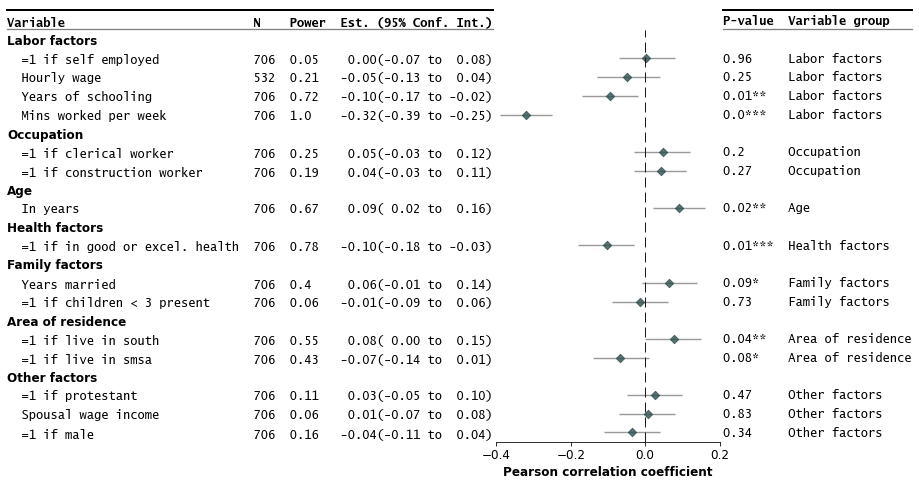This package makes publication-ready forest plots easy to make out-of-the-box. Users provide a `dataframe` (e.g. from a spreadsheet) where rows correspond to a variable/study with columns including estimates, variable labels, and lower and upper confidence interval limits. Additional options allow easy addition of columns in the `dataframe` as annotations in the plot.

• Installation

• Quick Start

• Some Examples with Customizations

• Gallery and API Options

• Known Issues

• Contributing

# Installation

```pip install forestplot
```
```git clone https://github.com/LSYS/forestplot.git
cd forestplot
pip install .
```

# Quick start

```import forestplot as fp

df = fp.load_data("sleep")  # companion example data
```

var

r

moerror

label

group

ll

hl

n

power

p-val

0

age

0.0903729

0.0696271

in years

age

0.02

0.16

706

0.671578

0.0163089

1

black

-0.0270573

0.0770573

=1 if black

other factors

-0.1

0.05

706

0.110805

0.472889

2

clerical

0.0480811

0.0719189

=1 if clerical worker

occupation

-0.03

0.12

706

0.247768

0.201948

(* This is a toy example of how certain factors correlate with the amount of sleep one gets. See the notebook that generates the data.)

The example input dataframe above have 4 key columns

Column

Description

Required

`var`

Variable field

`r`

Correlation coefficients (estimates to plot)

`moerror`

Conf. int.’s margin of error.

`label`

Variable labels

`group`

Variable grouping labels

`ll`

Conf. int. lower limits

✓*

`hl`

Containing the conf. int. higher limits

✓*

`n`

Sample size

`power`

Statistical power

`p-val`

P-value

(*If `ll` and `hl` are specified, then the `moerror` (margin of error) is not required.
See Gallery and API Options for more details on required and optional arguments.)

Make the forest plot

```fp.forestplot(df,  # the dataframe with results data
estimate="r",  # col containing estimated effect size
ll="ll", hl="hl",  # columns containing conf. int. lower and higher limits
varlabel="label",  # column containing variable label
ylabel="Confidence interval",  # y-label title
xlabel="Pearson correlation"  # x-label title
)
```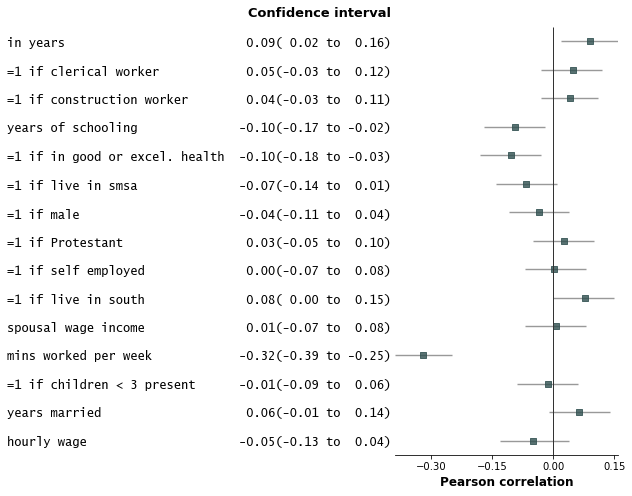# Some examples with customizations

1. Add variable groupings, add group order, and sort by estimate size.

```fp.forestplot(df,  # the dataframe with results data
estimate="r",  # col containing estimated effect size
moerror="moerror",  # columns containing conf. int. margin of error
varlabel="label",  # column containing variable label
# group ordering
group_order=["labor factors", "occupation", "age", "health factors",
"family factors", "area of residence", "other factors"],
sort=True  # sort in ascending order (sorts within group if group is specified)
)
```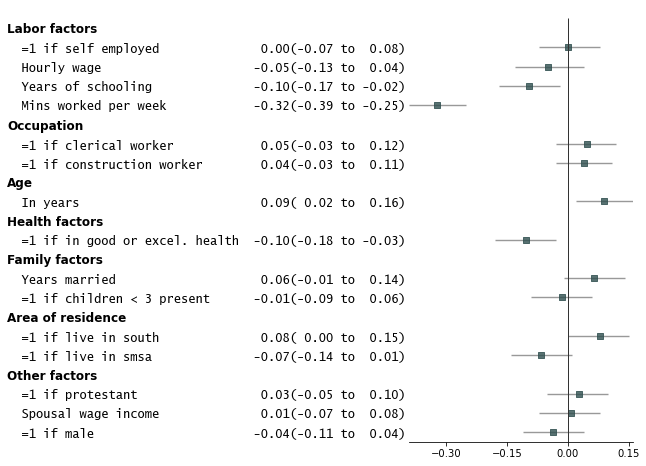1. Add p-values on the right and color alternate rows gray

```fp.forestplot(df,  # the dataframe with results data
estimate="r",  # col containing estimated effect size
ll="ll", hl="hl",  # columns containing conf. int. lower and higher limits
varlabel="label",  # column containing variable label
# group ordering
group_order=["labor factors", "occupation", "age", "health factors",
"family factors", "area of residence", "other factors"],
sort=True,  # sort in ascending order (sorts within group if group is specified)
pval="p-val",  # Column of p-value to be reported on right
color_alt_rows=True,  # Gray alternate rows
ylabel="Est.(95% Conf. Int.)",  # ylabel to print
**{"ylabel1_size": 11}  # control size of printed ylabel
)
```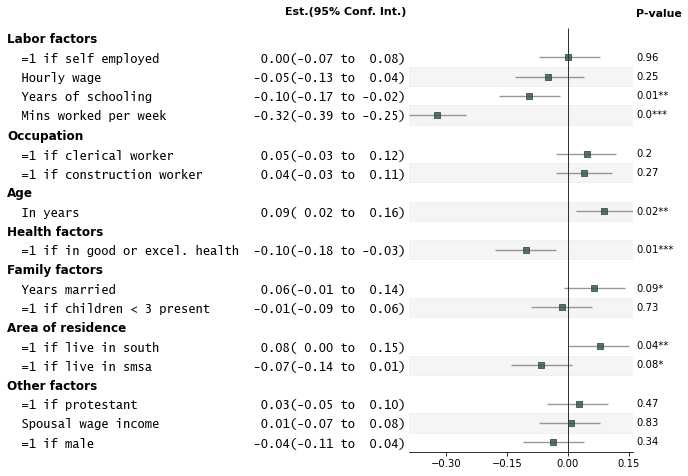1. Customize annotations and make it a table

```fp.forestplot(df,  # the dataframe with results data
estimate="r",  # col containing estimated effect size
ll="ll", hl="hl",  # lower & higher limits of conf. int.
varlabel="label",  # column containing the varlabels to be printed on far left
pval="p-val",  # column containing p-values to be formatted
annote=["n", "power", "est_ci"],  # columns to report on left of plot
rightannote=["formatted_pval", "group"],  # columns to report on right of plot
xlabel="Pearson correlation coefficient",  # x-label title
table=True,  # Format as a table
)
```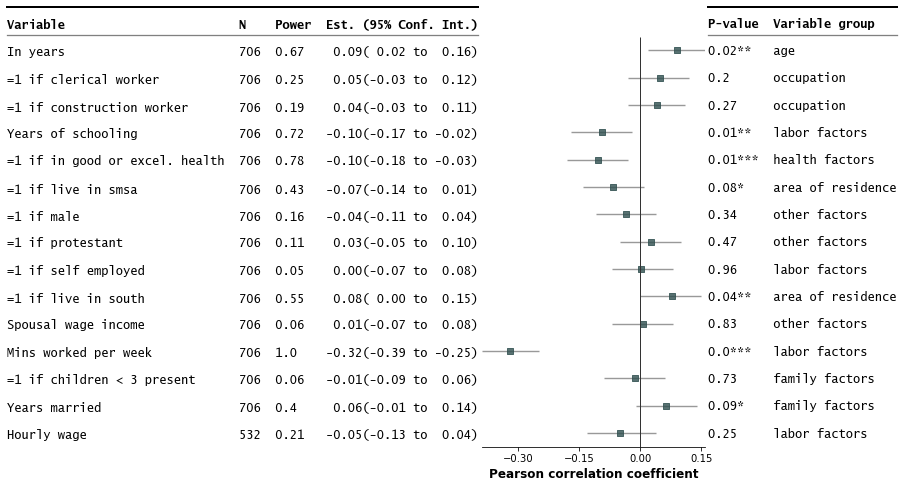1. Strip down all bells and whistle

```fp.forestplot(df,  # the dataframe with results data
estimate="r",  # col containing estimated effect size
ll="ll", hl="hl",  # lower & higher limits of conf. int.
varlabel="label",  # column containing the varlabels to be printed on far left
ci_report=False,  # Turn off conf. int. reporting
flush=False,  # Turn off left-flush of text
**{'fontfamily': 'sans-serif'}  # revert to sans-serif
)
```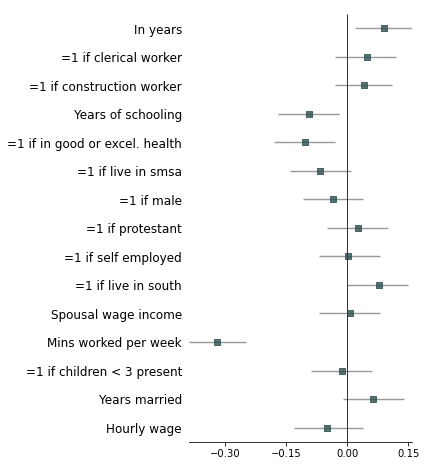1. Example with more customizations

```fp.forestplot(df,  # the dataframe with results data
estimate="r",  # col containing estimated effect size
ll="ll", hl="hl",  # lower & higher limits of conf. int.
varlabel="label",  # column containing the varlabels to be printed on far left
pval="p-val",  # column containing p-values to be formatted
annote=["n", "power", "est_ci"],  # columns to report on left of plot
rightannote=["formatted_pval", "group"],  # columns to report on right of plot
groupvar="group",  # column containing group labels
group_order=["labor factors", "occupation", "age", "health factors",
"family factors', "area of residence", "other factors"],
xlabel="Pearson correlation coefficient",  # x-label title
xticks=[-.4,-.2,0, .2],  # x-ticks to be printed
sort=True,  # sort estimates in ascending order
table=True,  # Format as a table
**{"marker": "D",  # set maker symbol as diamond
"markersize": 35,  # adjust marker size
"xlinestyle": (0, (10, 5)),  # long dash for x-reference line
"xlinecolor": ".1",  # gray color for x-reference line
"xtick_size": 12,  # adjust x-ticker fontsize
}
)
```Annotations arguments allowed include:
• `ci_range`: Confidence interval range (e.g. `(-0.39 to -0.25)`).

• `est_ci`: Estimate and CI (e.g. `-0.32(-0.39 to -0.25)`).

• `formatted_pval`: Formatted p-values (e.g. `0.01**`).

To confirm what processed `columns` are available as annotations, you can do:

```processed_df, ax = fp.forestplot(df,
...  # other arguments here
return_df=True  # return processed dataframe with processed columns
)
```

label

group

n

r

CI95%

p-val

BF10

power

var

hl

ll

moerror

formatted_r

formatted_ll

formatted_hl

ci_range

est_ci

formatted_pval

formatted_n

formatted_power

formatted_est_ci

yticklabel

formatted_formatted_pval

formatted_group

yticklabel2

0

Mins worked per week

Labor factors

706

-0.321384

[-0.39 -0.25]

1.99409e-18

1.961e+15

1

totwrk

-0.25

-0.39

0.0686165

-0.32

-0.39

-0.25

(-0.39 to -0.25)

-0.32(-0.39 to -0.25)

0.0***

706

1

-0.32(-0.39 to -0.25)

Mins worked per week 706 1.0 -0.32(-0.39 to -0.25)

0.0***

Labor factors

0.0*** Labor factors

1

Years of schooling

Labor factors

706

-0.0950039

[-0.17 -0.02]

0.0115515

1.137

0.72

educ

-0.02

-0.17

0.0749961

-0.1

-0.17

-0.02

(-0.17 to -0.02)

-0.10(-0.17 to -0.02)

0.01**

706

0.72

-0.10(-0.17 to -0.02)

Years of schooling 706 0.72 -0.10(-0.17 to -0.02)

0.01**

Labor factors

0.01** Labor factors

# Known Issues

• Variable labels coinciding with group variables may lead to unexpected formatting issues in the graph.

• Horizontal CI lines cannot be recast as capped horizontal lines because of the backend `Matplotlib` API used.

• Left-flushing of annotations relies on the `monospace` font.

• Plot can get cluttered with too many variables/rows (~30 onwards)

# Background and Additional Resources

Forest plots have many aliases (h/t Chris Alexiuk). Other names include coefplots, coefficient plots, meta-analysis plots, dot-and-whisker plots, blobbograms, margins plots, regression plots, and ropeladder plots.

Forest plots in the medical and health sciences literature are plots that report results from different studies as a meta-analysis. Markers are centered on the estimated effect and horizontal lines running through each marker depicts the confidence intervals.

The simplest version of a forest plot has two columns: one for the variables/studies, and the second for the estimated coefficients and confidence intervals. This layout is similar to coefficient plots (coefplots) and is thus useful for more than meta-analyses.

Here are more resources about forest plots:

•  Chang, Y., Phillips, M.R., Guymer, R.H. et al. The 5 min meta-analysis: understanding how to read and interpret a forest plot. Eye 36, 673–675 (2022).

•  Lewis S, Clarke M. Forest plots: trying to see the wood and the trees BMJ 2001; 322 :1479The package is lightweight, built on `pandas`, `numpy`, and `matplotlib`.

It is slightly opinioniated in that the aesthetics of the plot inherits some of my sensibilities about what makes a nice figure. You can however easily override most defaults for the look of the graph. This is possible via `**kwargs` in the `forestplot` API (see Gallery and API options) and the `matplotlib` API.

Planned enhancements include forest plots each row can have multiple coefficients (e.g. from multiple models).

Related packages:

•  [Stata] Jann, Ben (2014). Plotting regression coefficients and other estimates. The Stata Journal 14(4): 708-737.

•  [Python] Meta-Analysis in statsmodels

•  [Python] Matt Bracher-Smith’s Forestplot

•  [R] Solt, Frederick and Hu, Yue (2021) dotwhisker: Dot-and-Whisker Plots of Regression Results

•  [R] Bounthavong, Mark (2021) Forest plots. RPubs by RStudio

# Contributing

Contributions are welcome, and they are greatly appreciated!

Potential ways to contribute:

• Raise issues/bugs/questions

• Write tests for missing coverage

• Add features (see examples notebook for a survey of existing features)

• Add example datasets with companion graphs